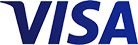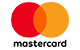Grid List
\$6.42 inc GST (Pair)
Increase value Decrease value
inc GST (Carton)
Increase value Decrease value
inc GST (Each)
Increase value Decrease value
inc GST (Each)
Increase value Decrease value
inc GST (Each)
Increase value Decrease value
inc GST (Carton)
Increase value Decrease value
inc GST (Carton)
Increase value Decrease value
inc GST (Each)
Increase value Decrease value
\$2.89 inc GST (Pair)
Increase value Decrease value
\$3.91 inc GST (Pair)
Increase value Decrease value
inc GST (Each)
Increase value Decrease value
inc GST (Pair)
Increase value Decrease value